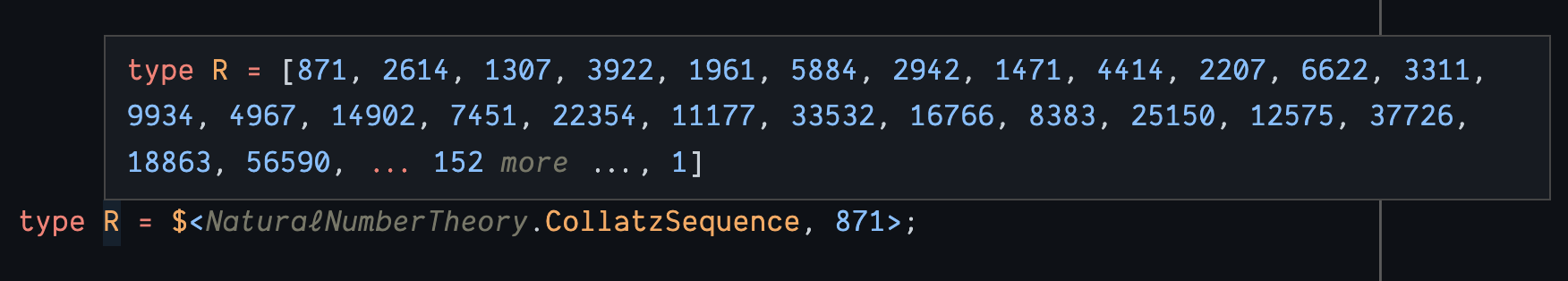# Type-level Collatz Sequence

The Collatz conjecture is an unsolved problem in mathematics. It states that for any positive integer n, the sequence of numbers generated by the following algorithm will eventually reach 1. These are also called the hailstone numbers.

function collatz(n: number): number {
return n % 2 === 0 ? n / 2 : 3 * n + 1;
}


The sequence generated by a given $n$ is the sequence of numbers n, collatz(n), collatz(collatz(n)), collatz(collatz(collatz(n))), and so on. This is known as the Collatz sequence of $n$, and ends when ‘1’ is reached.

## Typescript

This sequence serves as an entertaining challenge for performing type-level arithmetic, including addition, multiplication, and conditional logic.

Additionally, since even small numbers may generate large sequences, this serves as a benchmark for the performance and depth-limit of type-level computations.

## Method

The method I used was to compose various type-level operations into a single type-level function, which I called Collatz. This function takes a type N and returns the next element in the Collatz sequence of N.

import { $, Kind, Conditional, Function, NaturalNumber } from "hkt-toolbelt"; export type Collatz = Conditional.If< NaturalNumber.IsEven,$<NaturalNumber.DivideBy, 2>,
Kind.Pipe<[$<NaturalNumber.Multiply, 3>, NaturalNumber.Increment]> >;  These modules come from my library hkt-toolbelt. Essentially, these higher-order types compose to form a point-free programming language. All modules are highly optimized for performance and type inference. For generating the CollatzSequence, I used a fixed point combinator variant that I call FixSequence, which takes a homomorphic higher-order type$F$and an initial value$n$and returns the sequence$[n, F(n), F^2(n), F^3(n), …]$until the sequence reaches a fixed point (if it ever does). import {$, Kind, Combinator, Conditional, Function, NaturalNumber } from "..";

export type CollatzSequence = $< Combinator.FixSequence, Conditional.If<Conditional.Equals<1>, Function.Constant<1>, Collatz> >;  ## Results The screenshot below shows type inference for the Collatz sequence of$n = 871$, which has a sequence length of 179.The implementation even supports initial values into the millions: import {$, NaturalNumberTheory } from "hkt-toolbelt";

type R = \$<NaturalNumberTheory.CollatzSequence, 3_732_423>["length"]; // 597


## Access

The Collatz and CollatzSequence utilities are available in the NaturalNumberTheory module of hkt-toolbelt.